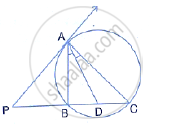Share

In the Figure; Pa is a Tangent to the Circle, Pbc is Secant and Ad Bisects Angle Bac. Show that Triangle Pad is an Isosceles Triangle. Also, Show That: ∠Cad =1/2(∠Pba-∠Pab) - ICSE Class 10 - Mathematics

ConceptTangent Properties - If a Line Touches a Circle and from the Point of Contact, a Chord is Drawn, the Angles Between the Tangent and the Chord Are Respectively Equal to the Angles in the Corresponding Alternate Segments

Question

In the figure; PA is a tangent to the circle, PBC is secant and AD bisects angle BAC. Show that triangle PAD is an isosceles triangle. Also, show that:

∠CAD =1/2(∠PBA-∠PAB)Solution

i) PA is the tangent and AB is a chord
∴ ∠PAB =  ∠C …….. (i) ( angles in the alternate segment)
AD is the bisector of ∠BAC
∴ ∠1 = ∠2 ……….(ii)
Ext. ∠ADP  = ∠C + ∠1
⇒ Ext ∠ADP =  ∠PAB + ∠2 = ∠PAD
Therefore, Δ PAD is an isosceles triangle.
ii) In ΔABC,
Ext. ∠PBA  =  ∠C  + ∠BAC
∠BAC =  ∠PBA  - ∠C
⇒ ∠1 + ∠2 = ∠PBA -  ∠PAB
(fom (i) part)

2∠1 = ∠PBA - ∠PAB

∠1=1/2(∠PBA - ∠PAB)

⇒∠CAD = 1/2 (∠PBA - ∠ PAB)

Is there an error in this question or solution?

Video TutorialsVIEW ALL 

Solution In the Figure; Pa is a Tangent to the Circle, Pbc is Secant and Ad Bisects Angle Bac. Show that Triangle Pad is an Isosceles Triangle. Also, Show That: ∠Cad =1/2(∠Pba-∠Pab)` Concept: Tangent Properties - If a Line Touches a Circle and from the Point of Contact, a Chord is Drawn, the Angles Between the Tangent and the Chord Are Respectively Equal to the Angles in the Corresponding Alternate Segments.
S